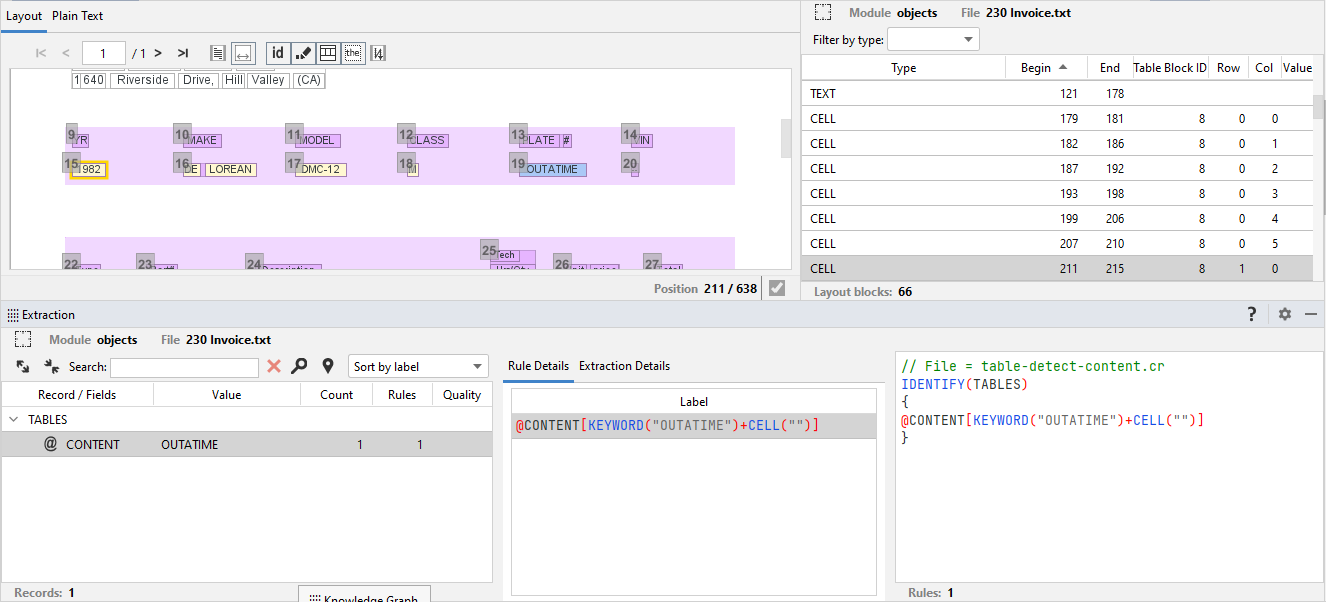# CELL attribute

The `CELL` attribute can be used in combination with other attributes to query and extract cells content from tables.

Tables in a document are detected if the document has been processed by the document understanding analysis with Extract.

Info

To know more about the smart document understanding analysis with Extract, read the expert.ai Studio user manual.

The syntax is:

`CELL("")`

or:

`CELL("r[n];c[m]")`

where:

• `r[n]` is the nth row of the table.
• `c[m]` is the mth column of the table.

To have a proper usage it is necessary specify the scope with the scope `TABLE`.

The first syntax is used to match the text that is contained in any cell of a table, while the second syntax is used to match the text in a cell located in a specific row and column.

For example to extract the value OUTATIME, if it is contained in a cell, the rule is:

``````SCOPE TABLE
{
IDENTIFY(TABLES)
{
@CONTENT[KEYWORD("OUTATIME")+CELL("")]
}
}
``````The `CELL` attribute arguments can be coordinates or range of coordinates expressed in columns and rows.

In the example below, the total_premium value field is extracted in column 2 (indices starting from 0), only if a cell at column 2 and row 0 (in the same table, given the constraint `SCOPE TABLE`) contains the text Total Premium.

``````SCOPE TABLE
{
IDENTIFY(POLICY)
{
@total_premium[CELL("r;c")] // extract text from cell row 4 and column 2
AND